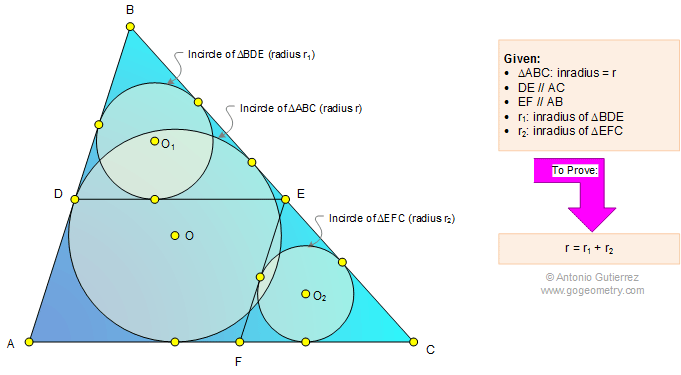Problem 1101: Triangle, Parallel Lines, Circle, Incircle, Inradius, Radius, Angle. Level: High School, SAT Prep, College Geometry

< PREVIOUS PROBLEM  |  NEXT PROBLEM >

 In a triangle ABC (see the figure below) DE // AC and EF //AB. If r, r1, and r2 are the inradii of triangles ABC, BDE, and EFC, respectively, prove that r = r1 + r2.Home | Search | Geometry | Problems | All Problems | Open Problems | Visual Index | 1101-1110 | Triangle | Circle | Incircle | Parallel lines | Similarity | Email | Post a comment or solution | By Antonio GutierrezLast updated: Mar 20, 2015